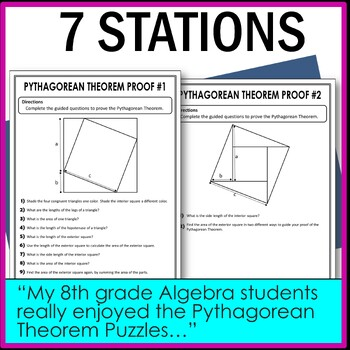# Pythagorean Theorem Stations Activity

Rated 5 out of 5, based on 3 reviews
3 Ratings;
8th - 9th, Homeschool
Subjects
Resource Type
Standards
Formats Included
• PDF
Pages
16 pages
Report this resource to TPT
##### Also included in
1. Looking to add variety to your classroom? These middle school math stations are just what you need for your students to develop a deep understanding of mathematics. Includes stations in the topics of number systems, expressions, equations, functions, ratios, proportional relationships, probability
Price \$67.00Original Price \$98.00Save \$31.00
2. These 8th grade math stations are just what you need to help your students develop a deep understanding of mathematics. These cover various topics such as the Pythagorean theorem, rational and irrational number, solving equations with variables on both sides, parallel lines with transversals and mo
Price \$13.50Original Price \$18.00Save \$4.50
3. Looking for ways to simplify planning while continuing to teach in-depth lessons? Then this 8th grade math curriculum and activities bundle is for you! This curriculum is aligned to the 8th grade common core standards. Each unit includes: guided notes, assessments/worksheets and activities such as:
Price \$200.00Original Price \$339.00Save \$139.00
4. These 8th grade math stations are just what you need to help your students develop a deep understanding of mathematics. There are at least four stations in each set. The focus of these stations is for students to discover and apply mathematical principles. The students will delve into the mathema
Price \$30.00Original Price \$42.00Save \$12.00
5. This Pythagorean Theorem unit includes notes, assessments, worksheets, activities and stations. This is perfect for your students to dive into the Pythagorean Theorem: proofs, solving for legs, solving for the hypotenuse, and real-world problems. In-depth notes to teach and fun activities to supplem
Price \$10.00Original Price \$16.00Save \$6.00
6. You are going to love these fun 8th grade math activities to supplement your curriculum. Stations, task cards, mystery pictures and more. These activities cover a variety of 7th grade math topics so that you can use them throughout the year. Check Out What's Included***BONUS FILE*** 8th Grade Math
Price \$90.00Original Price \$120.50Save \$30.50

### Description

These Pythagorean theorem stations will take your students to a new level of understanding. Students will learn proofs of the Pythagorean theorem, problem solve through puzzles, and even apply their knowledge to real life applications. These are great to review, reinforce and learn all about the Pythagorean theorem.

This product includes the following

• Pythagorean Theorem Proof #1 station - guides students through a proof
• Pythagorean Theorem Proof #2 station - more open-ended
• Pythagorean Puzzle #1 station
• Pythagorean Puzzle #2 station
• Pythagorean Theorem Application station
• Distances with Pythagorean Theorem station - using the coordinate plane
• Pythagorean Theorem with Radicals station
• Recording sheets

• Each stations will take about 15- 20 minutes to complete
• Great to differentiate teaching
• Great for group work as well
• Also could be used for homework

Other Products you may be Interested in

FOLLOW ME for more great products to make sense of math!

Remember that leaving feedback earns you points toward FREE TPT purchases!

Please feel free to contact me with any questions!

Michelle,

Make Sense of Math

makesenseofmath@yahoo.com

Total Pages
16 pages
Included
Teaching Duration
N/A
Report this resource to TPT
Reported resources will be reviewed by our team. Report this resource to let us know if this resource violates TPT’s content guidelines.

### Standards

to see state-specific standards (only available in the US).
Explain a proof of the Pythagorean Theorem and its converse.
Apply the Pythagorean Theorem to determine unknown side lengths in right triangles in real-world and mathematical problems in two and three dimensions.
Apply the Pythagorean Theorem to find the distance between two points in a coordinate system.